Name _____________________________Date ___________________
Similar Triangles
Complete.

 1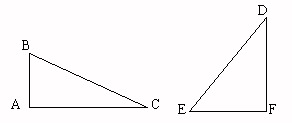m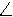BEmAFAC:FD = 6:12, and ED = 156, what is the length of BC?
 2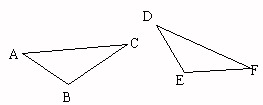mCFmADThe perimeter of smaller triangle ABC is 56. The lengths of two corresponding sides on the triangles are 23 and 138. What is the perimeter of DEF?
 3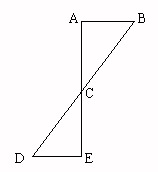mBEThe length of the sides of ABC are 175, 203, and 210. The length of the smallest side of DEC is 50, what is the length of the longest side of DEC?
 4mAFmBEThe length of the sides of ABC are 88, 156, and 100. The perimeter of FED is 172, what is the length of the longest side of FED?
 5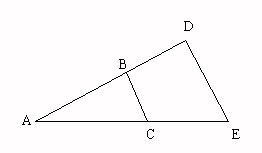mCEmBDThe perimeter of smaller triangle ABC is 80. The lengths of two corresponding sides on the triangles are 33 and 165. One side of ADE is 105. What is the length of the corresponding side on ABC?
 6mBEThe perimeter of smaller triangle ABC is 117. The lengths of two corresponding sides on the triangles are 44 and 220.
 7mAFmBEThe length of the sides of ABC are 60, 74, and 78. The length of the longest side of FED is 468, what is the perimeter of FED?
 8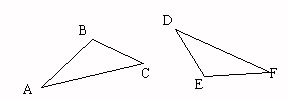mBEmADThe perimeter of smaller triangle ABC is 134. The lengths of two corresponding sides on the triangles are 48 and 240. One side of DEF is 235. What is the length of the corresponding side on ABC?
 9mAAmCEThe length of the sides of ABC are 220, 204, and 216. The length of the smallest side of ADE is 408, what is the length of the longest side of ADE?
 10mBEmAFThe perimeter of smaller triangle ABC is 183. The lengths of two corresponding sides on the triangles are 67 and 335.